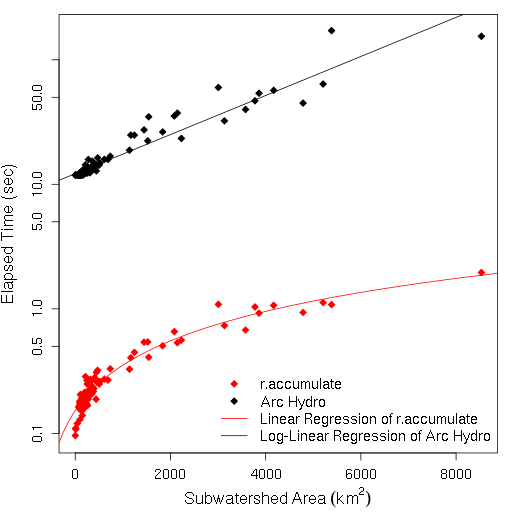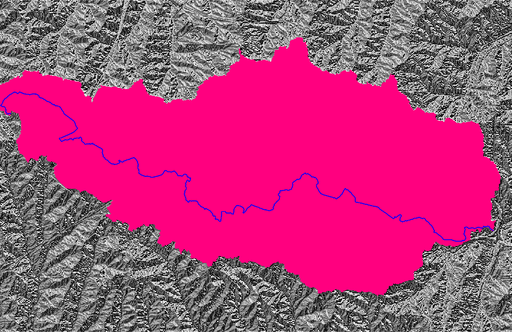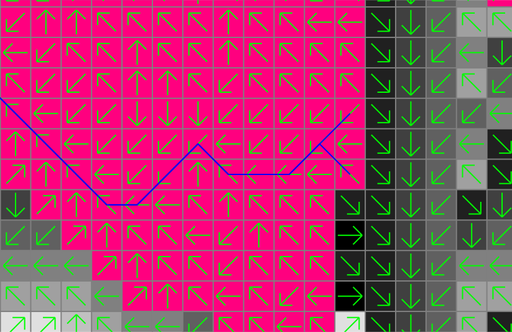Repository for Ideas & Research
Open Source GIS, Hydrologic Modeling, Optimization

# How to calculate the longest flow path in GRASS GIS

The r.accumulate module implements a recursive and its iterative algorithms for calculating the longest flow path based on Cho (2020). This module outperforms Arc Hydro’s Longest Flow Path tool.## 2   Deprecated raster approach

The r.stream.distance module can calculate the flow length downstream (FLDS) and flow length upstream (FLUS) raster maps. In the FLDS map, each cell has the flow length from that cell to the downstream-most outlet cell flowing outside the watershed. Outlet cells along the watershed boundary get assigned 0. Similarly, in the FLUS map, each cell has the flow length from that cell to the upstream-most cell where the watershed divide starts. Those cells along the watershed divide get assigned 0. These two raster maps have the same maximum value at the outlet cell in FLUS and the upstream-most cell on the longest flow path (LFP) in FLDS. If we add both maps, cells on the output map will have the same maximum value greater than non-LFP cells and we use that fact to calculate LFP.

This tutorial uses the North Carolina sample data set.

### 2.1   Calculate the longest flow path raster map

First, create the drainage direction map (drain_directions) from the elevation map (elevation).

``````g.region rast=elevation
r.watershed elevation=elevation drainage=drain_directions``````

Create the basin map (basin) at x=642455 and y=222614 and apply it as mask.

``````r.water.outlet input=drain_directions output=basin coordinates=642455,222614
g.region rast=basin

Create a raster map (outlet) containing the outlet point.

``````echo 642455,222614 | v.in.ascii input=- output=outlet separator=,
v.to.rast input=outlet output=outlet use=cat``````

Calculate FLDS (flds) and FLUS (flus).

``````r.stream.distance -o stream_rast=outlet direction=drain_directions method=downstream distance=flds
r.stream.distance -o stream_rast=outlet direction=drain_directions method=upstream distance=flus``````

Combine FLDS and FLUS to create a new raster map (fldsus).

``r.mapcalc expression="fldsus=flds+flus"``

Find the maximum flow length that each cell on FLDSUS got assigned.

``r.info -r map=fldsus | sed '/^max=/!d; s/^max=//'``

Create the LFP raster map (lfp) allowing small floating-point errors in FLDSUS. The maximum cell value obtained from the last step (or LFP distance) was 21452.825. Subtract a small number to avoid a potential floating-point error.

``r.mapcalc expression="lfp=if(fldsus>=21452.825-0.0005,1,null())"``

Now, lfp is the longest flow path raster map.

### 2.2   Calculate the longest flow path vector map

Simply converting the lfp raster map to vector won’t produce the correct vector map because of this issue. To properly trace the longest flow path in vector format, we need to find the headwater raster cells and start tracing flow directions from there. Using the maximum flow length found above (21452.825), find the headwater cells from FLDS and create a new vector map with the headwater points.

``````r.mapcalc expression="heads=if(flds>=21452.825-0.0005,1,null())"

Trace flow directions from the headwater points to calculate the longest flow path in vector format.

``r.path input=drain_directions vector_path=path start_points=heads``

The output vector map (path) includes the longest flow path, but it passes through the outlet and flows beyond the watershed. We need to split the path at the outlet first and select only the upstream segment within the watershed. To split the path at the outlet, create a new vector map with the snapped outlet point.

``r.to.vect input=outlet output=outlet type=point``

Find the coordinates of the snapped outlet. Read snapped x=642455 and y=222615 and split the path at this point.

``````v.to.db -p map=outlet option=coor
v.edit map=path tool=break coords=642455,222615``````

Select the upstream segment only that touches the headwater points.

``v.select ainput=path binput=heads output=lfp``

Finally, we got the longest flow path in lfp.This example produces two lines with the same flow length.